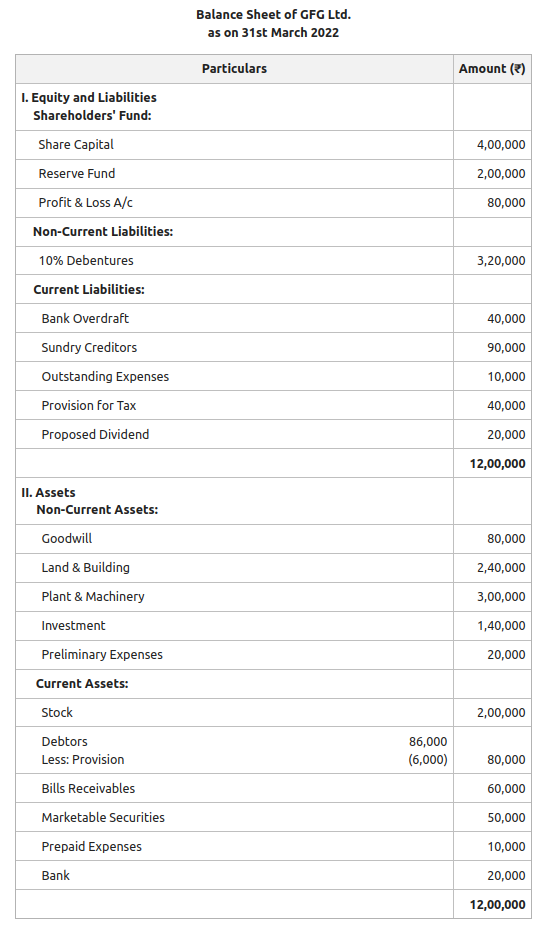GFG App
Open AppBrowser
Continue

# Current Ratio: Meaning, Significance and Examples

The ratio that is used to derive a relation between the current assets and current liabilities of a firm is called a Current Ratio. It is used to determine whether the current assets of a firm would be sufficient to pay off its current obligations or not. In other words, it is used to depict the magnitude of current assets against current liabilities of a concern. It is also known as Working Capital Ratio.

A Current Ratio is one of the two main liquidity ratios. Liquidity ratios are the ones that determine the organisation’s ability to meet its short-term obligations by defining a systematic relationship between the amount of current and/or liquid assets and that of the current/ short-term obligations.

Generally, a current ratio of 2:1 is considered ideal, which means that the current assets must be twice the amount of current liabilities. It is noteworthy that an organisation may or may not have this ideal ratio at all points in an accounting period. But it must try its best to maintain the ideal ratio to ensure liquidity and creditworthiness.

Current Assets and Current Liabilities can be defined as:

• Current Assets: Such assets which are held by an enterprise to be converted into cash within a period of one year or less through standard business operations are called current assets. In other words, current assets are not held for the purpose of capitalisation.  Examples: Cash, Cash Equivalents, Stock or Inventory, Accounts Receivable, Marketable Securities, Prepaid Expenses, etc.
• Current Liabilities: This refers to the amounts payable to the firm’s creditors and lenders within a period of one year or within the standard operating cycle, whichever is shorter. They are typically settled using the firm’s current assets. Examples: Accounts payable, short-term debts like commercial paper, current maturity of long-term loans, income tax due for the year, etc.

### Formula:Where,

Current Assets = Cash in Hand + Cash at Bank + Short-term Investments (Marketable Securities) + Trade Receivables (Less Provision) + Inventories (Stock of Finished Goods + Stock of Raw Material + Work in Progress) + Prepaid Expenses

Current Liabilities = Bank Overdraft + Trade Payables + Provision of Taxation + Proposed Dividends + Unclaimed Dividends + Outstanding Expenses + Loans Payable within a Year

#### There can be three situations arising from the calculation:

If Current Assets > Current Liabilities, then Current Ratio > 1: This implies that the organisation would still have some assets left even after paying all the short-term debts and is a desirable situation to be in.

If Current Assets = Current Liabilities, then Current Ratio = 1:  This means that the current assets are just enough to cover the short-term obligations of the firm.

If Current Assets < Current Liabilities, then Current Ratio < 1This is not an ideal situation to be in since it implies that the company does not have enough resources to pay off short-term debts.

### Significance:

Current Ratio is computed to know the ability of a firm to pay off the short-term liabilities of a firm with the help of current assets. It is assumed that all the current assets are likely to be converted into cash to pay off the short-term liabilities of the firm. In other words, this ratio is calculated to determine the short-term solvency of a firm. 2:1 is considered an ideal current ratio. That means the current assets should be double the current liabilities of the firm. But if the current ratio is very high, it is believed that the funds are lying idle and the firm has poor control over its inventory or debtors turnover is slow.

Illustration 1:

Calculate the current ratio from the following Balance Sheet for GFG Ltd. for the year ending March 2022 and comment on the result.Solution:Current Assets = Stock + Debtors + Bills Receivables + Marketable Securities+  Prepaid Expenses + Bank

= 2,00,000 + 80,000 + 60,000 + 50,000 + 10,000 + 20,000

= ₹4,20,000

Current Liabilities = Bank Overdraft + Sundry Creditors + Outstanding Expenses + Provision for Tax + Proposed Dividend

= 40,000 + 90,000 + 10,000 + 40,000 + 20,000

= ₹2,00,000

Therefore,= 2.1 : 1

Comment: The current ratio of Geeks Ltd. is 2.1:1, which is slightly more than the ideal ratio. It implies that the short-term financial position of the firm is sound, and it can meet its short-term liabilities well in time.

Illustration 2:

Calculate Current Ratio from the following Information:

Total Debt = ₹1,00,800; Long-term Debt = ₹60,400 and Working Capital = ₹50,000

Solution:

Current Liabilities = Total Debt – Long-term Debt

= 1,00,800 – 60,400

= ₹40,400

Working Capital = Current Assets – Current Liabilities

50,000 = Current Assets – 40,400

50,000 + 40,400 = Current Assets

Therefore, Current Assets = ₹90,400= 2.24 : 1

My Personal Notes arrow_drop_up